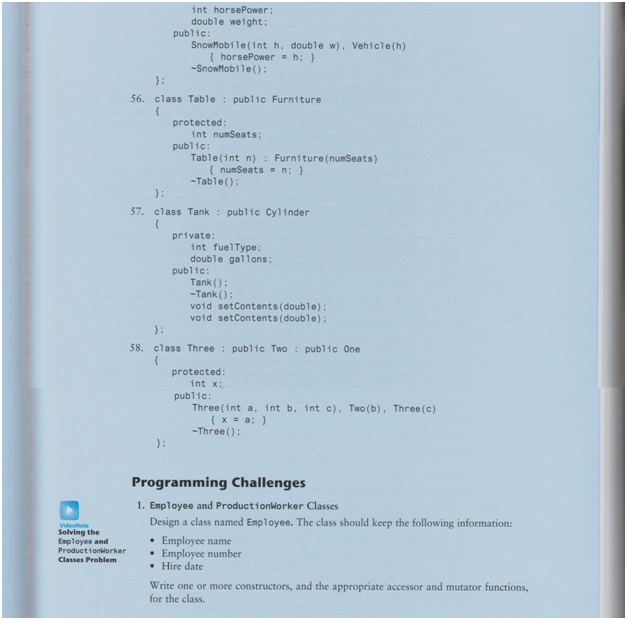# The best Parser scanner assignment help service

We offer a high-quality parser scanner assignment help services. Our services are available on a 24/7 basis and are pocket-friendly for students worldwide. Our first-class Parser scanner assignment help has been made possible by the significant number of experts at our disposal. Our objective is to ensure that you get excellent solutions at the right time. Therefore, do not worry about late deliveries. What is more, when you seek our assignment help class, you enjoy good students' discounts. Do not struggle with your assignment when you can get the best help from us at an affordable price.

## Fibonacci function/Subroutine Using C Programming Language

In this sample C programming assignment a subroutine asuAdd is given. Students are required to write a function in C Programming language using the subroutine asuAdd to calculate huge Fibonacci numbers.

The prototype/signature for the function is given as follows : –n is the Fibonacci number that needs to be calculated. The series of Fibonacci number starts from values 0, 1; and n is to be calculated.

#### SOLUTION : –

#include

#define MAX_SIZE_TEST 1000

typedef unsigned int bigNumTest[1 + MAX_SIZE_TEST];

typedef unsigned int bigNumN[];

int asuAdd(bigNumN bigN0P, const bigNumN bigN1P, unsigned int maxN0Size);

void halt()

{

}

/*

* function: asuFibonacci

*

* n is the Fibonacci number that you would like to calculate. (Our Fibonacci

* numbers start with f0 = 0, f1 = 1, etc., and we would like to calculate fn.)

*

* The return value of the function is either n, or the largest Fibonacci number

* that could be accurately calculated before overflow occurred.

*

* maxSize is an integer that specifies how many valid words can be stored in the

* bigNumN numbers.

*

* bNP is a pointer to a pointer to a bigNumN number holding the Fibonacci number

* indicated by the return value. bNP will either point to static array bNa or

* static array bNb.

*

*/

int asuFibonacci(int n, int maxSize, bigNumN **bNP) {

// **** modify below and fill in code and ensure proper operation including return value. ***

static bigNumTest bNa = {1,0,0}; // initial fibonacci (0) = 0

static bigNumTest bNb = {1,1,0}; // initial fibonacci (1) = 1

int i;

if (n == 0)

{

*bNP = &bNa;

return 0;

}

else if (n == 1)

{

*bNP = &bNb;

return 1;

}

*bNP = &bNb;

for (i = 2; i <= n; i++)

{

if (!(i % 2)) // If it’s even

{

{

// if overflow, return last i

n = i – 1;

break;

}

*bNP = &bNa;

}

else // if it’s odd

{

{

// if overflow, return last i

n = i – 1;

break;

}

*bNP = &bNb;

}

}

return n;

}

int main() {

bigNumN *fibResP = NULL;

int i = asuFibonacci(1000000, MAX_SIZE_TEST, &fibResP);

halt();

return i;

}

// or move this to the assembly file

void _exit(int status) {

volatile int x; // work around problem with xilinx system debugger.

loop:

x++;

goto loop;

}